# Calculations

Calculations can be used to transform the data that is visualized.

## Creating a calculation

Add a chart to the process view and click the '...' button to the right of series in the properties: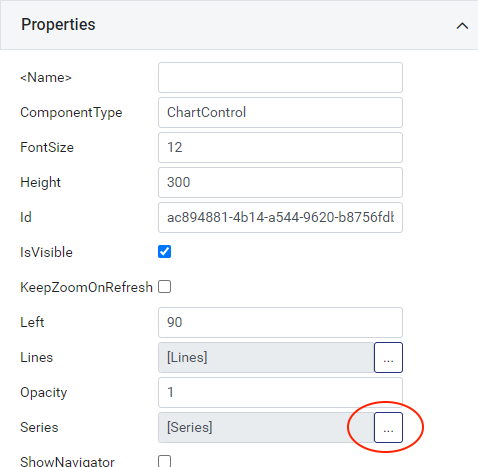You can then add multiple series to the chart. Click the data-bind button for the data on the first series: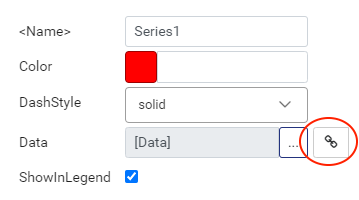Select calculation under source type: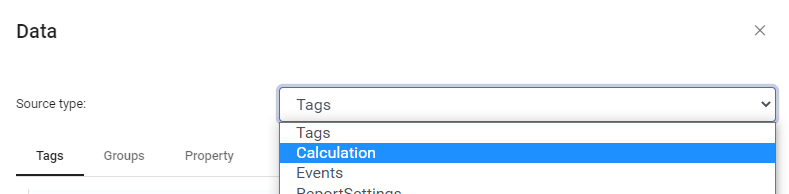You will see the following fields: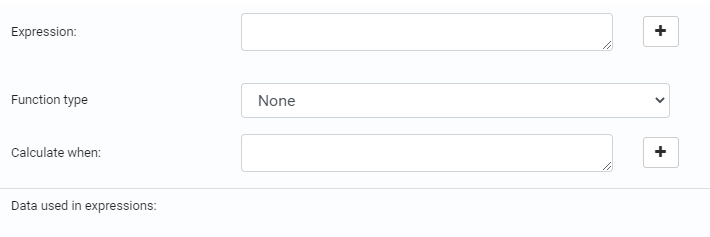The expression field is the calculation expression.

Function type is used to apply an aggregation function to the calculation.

Calculate when is used to filter the data. For example you could select time as the function type and add an is running condition in this field to calculate the run time of your machine.

For now click the +-icon to the right of the expression field and select a tag. Then type a + character in the expression and click the '+' and select another tag. You should end up with this: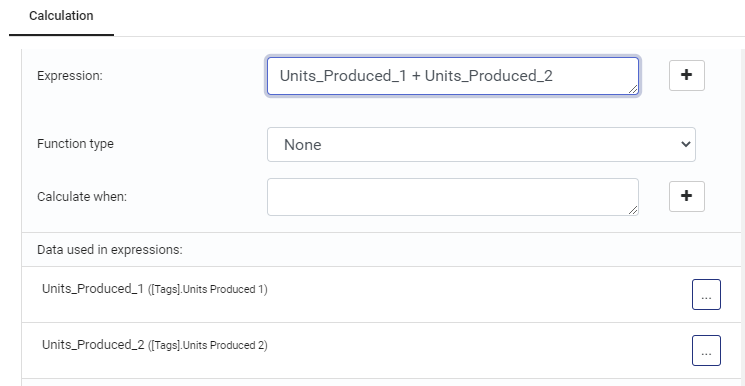Close the dialogs by clicking OK and you should now have a chart displaying the sum of two tags in a series.

## Single values compared to multiple values

Some calculations will result in a single value and some will result in multiple values (a series). This depends on the function type that is selected. It is important to keep this in mind and to select a suitable control for the value that you're visualizing.

In the example above there is no function type, so the calculation will calculate the sum of the two values every time either of the values change.

When selecting a function type, for example Maximum or Time, the result is only a single value which is not suitable to display in a chart control. Those values can be bound to for example a text control or gauge control instead.

It is also possible to apply a grouping function (for example grouping by time) so that a function can result in multiple values, one for each time group.

## Examples

### Machine runtime

Binding the following to a text control gives you the run time of a machine.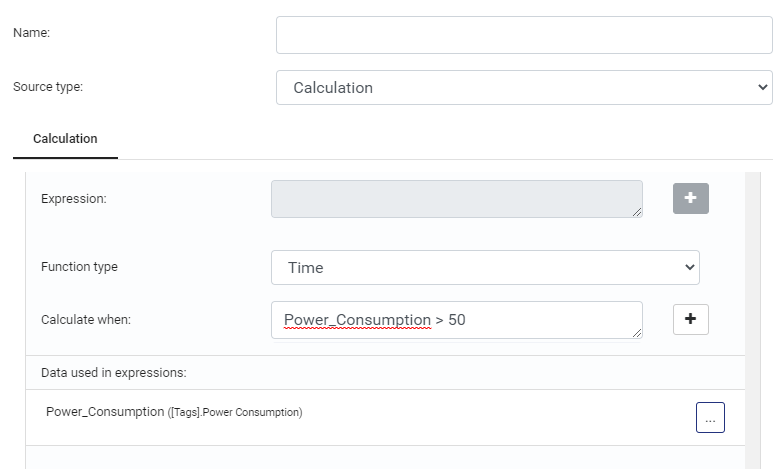This will show you the time (in hours) that the power consumption was above 50.